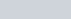A man arranges to pay off a dept of Rs 3600 by 40 annual instalments which form an
Question:

A man arranges to pay off a dept of Rs 3600 by 40 annual instalments which form an arithmetic series. When 30 of the instalments are paid, he dies leaving one-third of all debt unpaid, find the value of the first instalment.

Solution:

In the given problem,

Total amount of debt to be paid in 40 installmentsAfter 30 installments one−third of his debt is left unpaid. This means that he paid two third of the debt in 30 installments. So,

Amount he paid in 30 installments $=\frac{2}{3}(3600)$

$=2(1200)$

$=2400$

Let us take the first installment as and common difference as d.

So, using the formula for the sum of n terms of an A.P,

$S_{n}=\frac{n}{2}[2 a+(n-1) d]$

Let us find and d, for 30 installments.

$S_{30}=\frac{30}{2}[2 a+(30-1) d]$

$2400=15[2 a+(29) d]$

$\frac{2400}{15}=2 a+29 d$

$160=2 a+29 d$

$a=\frac{160-29 d}{2}$ ……(1)

Similarly, we find $a$ and $d$ for 40 installments.

$S_{40}=\frac{40}{2}[2 a+(40-1) d]$

$3600=20[2 a+(39) d]$

$\frac{3600}{20}=2 a+39 d$

$180=2 a+39 d$

$a=\frac{180-39 d}{2}$…………..(2)

Subtracting (1) from (2), we get,

$a-a=\left(\frac{180-39 d}{2}\right)-\left(\frac{160-29 d}{2}\right)$

$0=\frac{180-39 d-160+29 d}{2}$

$0=20-10 d$

Further solving for d,

$10 d=20$

$d=\frac{20}{10}$

$d=2$

Substituting the value of d in (1), we get.

$a=\frac{160-29(2)}{2}$

$=\frac{160-58}{2}$

$=\frac{102}{2}$

$=51$

Therefore, the first installment is Rs 51 .